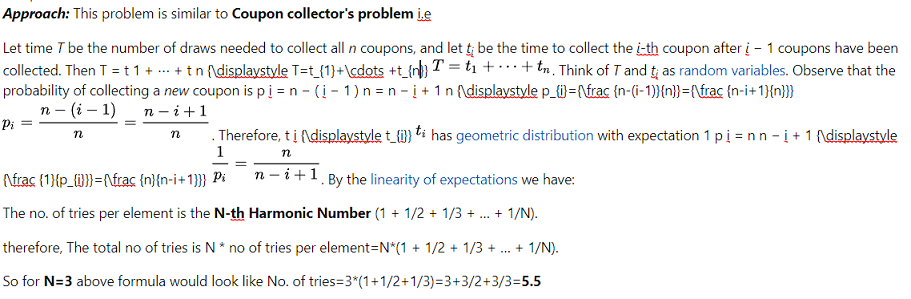# Expected Number of Trials until Success

• Difficulty Level : Medium
• Last Updated : 25 May, 2022

Consider the following famous puzzle.
In a country, all families want a boy. They keep having babies till a boy is born. What is the expected ratio of boys and girls in the country?
This puzzle can be easily solved if we know following interesting result in probability and expectation.
If probability of success is p in every trial, then expected number of trials until success is 1/p
Proof: Let R be a random variable that indicates number of trials until success.

```The expected value of R is sum of following infinite series
E[R] = 1*p + 2*(1-p)*p + 3*(1-p)2*p + 4*(1-p)3*p + ........

Taking 'p' out
E[R] = p[1 + 2*(1-p) + 3*(1-p)2 + 4*(1-p)3 + .......] ---->(1)

Multiplying both sides with '(1-p)' and subtracting
(1-p)*E[R] = p[1*(1-p) + 2*(1-p)2 + 3*(1-p)3 + .......] --->(2)

Subtracting (2) from (1), we get

p*E[R] = p[1 + (1-p) + (1-p)2 + (1-p)3 + ........]

Cancelling p from both sides
E[R] = [1 + (1-p) + (1-p)2 + (1-p)3 + ........]

Above is an  infinite geometric  progression with ratio (1-p).
Since (1-p) is less than, we can apply sum formula.
E[R] = 1/[1 - (1-p)]
= 1/p```

Solution of Boys/Girls ratio puzzle:
Let us use the above result to solve the puzzle. In the given puzzle, probability of success in every trial is 1/2 (assuming that girls and boys are equally likely).

```Let p be probability of having a baby boy.
Number of kids until a baby boy is born = 1/p
= 1/(1/2)
= 2
Since expected number of kids in a family is 2,
ratio of boys and girls is 50:50. ```

Let us discuss another problem that uses above result.
Coupon Collector Problem 1:
Suppose there are n types of coupons in a lottery and each lot contains one coupon (with probability 1 = n each). How many lots have to be bought (in expectation) until we have at least one coupon of each type.
The solution of this problem is also based on above result.
Let Xi be the number of lots bought before i’th new coupon is collected.
Note that X1 is 1 as the first coupon is always a new coupon (not collected before).
Let ‘p’ be probability that 2nd coupon is collected in next buy. The value of p is (n-1)/n. So the number of trials needed before 2nd new coupon is picked is 1/p which means n/(n-1). [This is where we use above result]
Similarly, the number of trials needed before 3rd new coupon is collected is n/(n-2)

```Using Linearity of expectation,
we can say that the total number of expected trials =
1 + n/(n-1) + n/(n-2) + n/(n-3) + .... + n/2 + n/1
= n[1/n + 1/(n-1) + 1/(n-2) + 1/(n-3) + ....+ 1/2 + 1/1]
= n * Hn
Here Hn is n-th Harmonic number

Since Logn <= Hn <= Logn + 1, we need to buy around
nLogn lots to collect all n coupons. ```

Coupon Collector Problem 2: (Approach and implementation with an Example):

There are N elements in an array. In the very beginning, random element is chosen. After some element are over, the next one is chosen randomly and independently of what have been selected before. Find the minimum selections to be made until every element is selected at least once.

Note: Answer will be considered as correct if it has an absolute or relative error less than 10^(-1). More formally if the expected output is A and your output is B, your output will be considered as correct if and only if |A − B| ≤ 10−1 * max{|A|, |B|, 1}.

Examples:

Input:
Output: 1.0
Explanation: There is only one element and it needs to be selected to make all elements as selected.

Input: 2
Output: 3.0
Explanation: After selecting the first element, there is 1/2 chance to finish the array each time new element is selected. So the expected number of elements selected is 2/2 + 3/4 + 4/8… = 3.

Input: 3
Output: 5.5
Explanation: Elements selected are 3/1+3/2+3/3=5.5Follow the steps to solve the problem:

1. Initialize ans (no. of tries) to 0.
2. Traverse from 1 to N by i.
3. Add consecutive values of N / i to ans.
4. Return ans as the final answer.

Below is the implementation of the above approach:

## C++14

 `//C++ code for the above approach:`   `#include ` `using` `namespace` `std;`   `// Function to find minimum selections` `double` `minSelections(``int``& n){` `   ``double` `ans = 0;` `   ``for``(``int` `i = 1; i <= n; i++)` `       ``ans += 1.0 * n / i;` `        `  `   ``return` `ans;` `}`   `// Driver's code` `int` `main() {` `int` `N = 3;` `cout << fixed << setprecision(8) << minSelections(N);` `return` `0;` `}`

## Java

 `// JAVA program of th above approach` `import` `java.util.*;` `class` `GFG` `{`   `// Function to find minimum selections` `static` `double` `minSelections(``int` `n){` `   ``double` `ans = ``0``;` `   ``for``(``int` `i = ``1``; i <= n; i++)` `       ``ans += ``1.0` `* n / i;` `         `  `   ``return` `ans;` `}`   `// Driver Code` `public` `static` `void` `main(String[] args)` `{` `    ``int` `N = ``3``;` `    ``System.out.print(String.format(``"%.8f"``, minSelections(N)));` `}` `}`   `// This code is contributed by code_hunt.`

Output

`5.50000000`

Time Complexity: O(N)
Auxiliary Space: O(1)

Exercise:
1) A 6 faced fair dice is thrown until a ‘5’ is seen as result of dice throw. What is the expected number of throws?
2) What is the ratio of boys and girls in above puzzle if probability of a baby boy is 1/3?
Reference:
http://www.cse.iitd.ac.in/~mohanty/col106/Resources/linearity_expectation.pdf
http://ocw.mit.edu/courses/electrical-engineering-and-computer-science/6-042j-mathematics-for-computer-science-fall-2010/video-lectures/lecture-22-expectation-i/